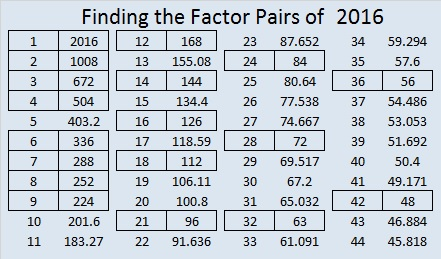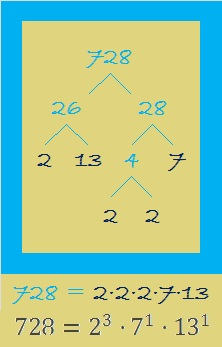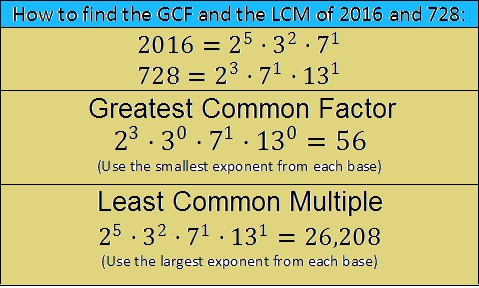# 728 Number Facts and Factors of the Year 2016

The year 2016 will have some fun mathematical properties:

2016 is the sum of all the counting numbers from 1 to 63. That makes 2016 the 63rd triangular number which can be calculated rather quickly using 63 x 64/2 = 2016. That is definitely an example of multiplication being a shortcut for addition!

When was the last time a year was a triangular number? 1953. That’s the sum of the first 62 numbers, and it is also the year that I was born.

Besides being the sum of the counting numbers from 1 to 63, 2016 is the sum of consecutive numbers a few other ways as well:

• 671 + 672 + 673 = 2016; that’s 3 consecutive numbers.
• 285 + 286 + 287 + 288 + 289 + 290 + 291 = 2016; that’s 7 consecutive numbers.
• 220 + 221 + 222 + 223 + 224 + 225 + 226 + 227 + 228 = 2016; that’s 9 consecutive numbers.
• 86 + 87 + 88 + 89 + 90 + 91 + 92 + 93 + 94 + 95 + 96 + 97 + 98 + 99 + 100 + 101 + 102 + 103 + 104 + 105 + 106 = 2016; that’s 21 consecutive numbers.

2016 is the sum of the eighteen prime numbers from 71 to 157.2016 is also the 32nd hexagonal number because 2 x 32² – 32 = 2016. (All hexagonal numbers are also triangular numbers, and half of all triangular numbers are hexagonal numbers.)

—————–

As you read this post, you may get the impression that 2016 is a perfect number. All perfect numbers are triangular numbers AND hexagonal numbers, but 2016 is still NOT a perfect number. Here’s why:

The sum of the first 31 factors of 2016 is

1 + 2 + 3 + 4 + 6 + 7 + 8 + 9 + 12 + 14 + 16 + 18 + 21 + 24 + 28 + 32 + 36 + 56 + 63 + 72 + 84 + 96 + 112 + 126 + 144 + 168 + 224 + 252 + 288 = 2016

but if you add in its other four factors 336 + 504 + 672 + 1008, you get 4536 which is way too much to make 2016 a perfect number.

True, 2016 is (2^5)(2^6 – 1) = 32 x 63, and all perfect numbers can be written similarly with consecutive powers of 2, BUT the odd factor, 63, is not a PRIME number so 2016 is not a perfect number.

IF 63 WERE a prime number, then the sum of the factors of 2016 would be

1 + 2 + 4 + 8 + 16 + 32 + 63 + 126 + 252 + 504 + 1008 = 2016

But again, 63 is NOT a prime number so 3, 6, 7, 9, 12, 14, 18, 21, 24, 28, 36, 42, 48, 56, 72, 84, 96, 112, 144, 168, 224, 288, 336, and 672 are also factors of 2016, and 2016 is NOT a perfect number.

—————–

Even though 2016 is NOT a perfect number, it is still an exciting number in many more ways:

2016 = (10 × 9 × 8 × 7 × 6)/(5 + 4 + 3 + 2 + 1)

From Stetson.edu we learn that the sum of the square and cube of 2016 is a number containing all the digits 0 – 9 exactly once:2016 is the short leg in these four primitive Pythagorean triples:

• 2016-3713-4225
• 2016-12,463-12,625
• 2016-20,687-20,785
• 2016-1,016,063-1,016,065

2016 is not a palindrome in any base until base 47. We just need a symbol to represent 42 base 10 in base 47 because 42(47) + 42(1) = 2016.

Thank you Slate magazine  for including my post in an article about properties of 2016. Also thank you for referring me to eljjdx.canalblog.com which has some very interesting information about the number 2016 that I didn’t include in this post. Something on my computer did a great job translating both of those articles from French into English.

Reflexivemaths has also written many thoughtful starter questions that explore the number 2016.

Before I give all the factors we can expect to see in the year 2016, let me share a few fun number facts that I saw on twitter:

//platform.twitter.com/widgets.js

//platform.twitter.com/widgets.js

//platform.twitter.com/widgets.js

//platform.twitter.com/widgets.js

//platform.twitter.com/widgets.js

//platform.twitter.com/widgets.js

//platform.twitter.com/widgets.js

//platform.twitter.com/widgets.js

That is the most complicated use of combinations I have ever seen. The innermost parenthesis means 4!/(2!∙2!) which equals 6. Since 2 was raised to that power, we get 2^6 = 64. Then 64!/(62!∙2!) = 2016.

//platform.twitter.com/widgets.js

//platform.twitter.com/widgets.js

————————————————

The last two years on New Year’s Eve I have predicted the factors of the coming year. Each time my predictions were 100% accurate. I am now ready to make my predictions for the factors of 2016:

(Drum roll) There will be 36 positive factors for 2016:

1, 2, 3, 4, 6, 7, 8, 9, 12, 14, 16, 18, 21, 24, 28, 32, 36, 56, 63, 72, 84, 96, 112, 126, 144, 168, 224, 252, 288, 336, 504, 672, 1008, 2016. Sadly, if you multiply any of them by -1, you’ll know a negative factor for 2016, too.

The factors of 2016 will also come in pairs: 1 x 2016, 2 x 1008, 3 x 672, 4 x 504, 6 x 336, 7 x 288, 8 x 252, 9 x 224, 12 x 168, 14 x 144, 16 x 126, 18 x 112, 21 x 96, 24 x 84, 28 x 72, 32 x 63, 36 x 56and 42 x 48.Because the number of 2016’s prime factors is a power of two, 2016 can make a nicely proportioned factor tree especially if we use any of the factor pairs that are in red. (14 x 114 is in bold only because I like the way it looks.) Because 2016 has 8 prime factors, we can get a very full and impressive tree. For example:Since this is my 728th post, I’ll write a little about the number 728, too. I’ll start with this factor tree for 728:It’s not as impressive as the factor tree for 2016, but did you notice that 2016 and 728 share several of the same prime factors?

When two numbers share some of the same factors, we may wonder what is the greatest common factor and what is the least common multiple?

One way to find either value is to use the prime factorization of both numbers. The prime factorization of 2016 uses three bases: 2, 3, and 7, while the prime factorization for 728 uses these bases: 2, 7, and 13. Write down all those bases without duplication, and you get 2, 3, 7, and 13.

For the GREATEST COMMON FACTOR (GCF), look at the prime factorizations and choose the SMALLEST exponent that appears in each. The greatest common factor cannot be bigger than the smallest number, 728. In this case it is 56 which will divide evenly into both 728 and 2016.For the LEAST COMMON MULTIPLE (LCM), look at the prime factorizations and choose the LARGEST exponent that appears in each. The least common multiple cannot be smaller than the largest number, 2016. In this case it is 26,208 which both 728 and 2016 can divided into evenly.

This method for finding the GCF and LCM will also work for three, four, or more numbers and even variable bases like x, y, or z. I encourage you to give it a try!

Here are some more number facts about the number 728:

26 x 28 = 728, and both 26 and 28 are one number away from 27, their average, so 728 is one number away from 27².

Here’s proof: 26 x 28 = (27 – 1)(27 + 1) = (27² – 1²) = 729 – 1 = 728.

Because 13 is one of its prime factors, 728 is the hypotenuse of Pythagorean triple 280-672-728. The greatest common factor of those three numbers is the same as the greatest common factor of 728 and 2016, but what is their least common multiple? It turns out to be 5 x 12 x 13 x 56, the product of the numbers in the primitive Pythagorean triple, 5-12-13, and 56. Using prime factorizations we get (2^5)(3^1)(5^1)(7^1)(13^1). We get the same answer using either method.

728 is a palindrome (repdigit) in three other number bases:

• 728 is 222222 BASE 3; note that 2(3^5) + 2(3^4) + 2(3^3) + 2(3^2) + 2(3^1) + 2(3^0) = 728.
• 888 BASE 9; note that 8(81) + 8(9) + 8(1) = 728.
• QQ BASE 27 (Q = 26 base 10); note that 26(27) + 26(1) = 728.

Here is more factoring information for 728:

————————————————

• 728 is a composite number.
• Prime factorization: 728 = 2 x 2 x 2 x 7 x 13, which can be written 728 = (2^3) x 7 x 13
• The exponents in the prime factorization are 1, 3, and 1. Adding one to each and multiplying we get (1 + 1)(3 + 1)(1 + 1) = 2 x 4 x 2 = 16. Therefore 728 has exactly 16 factors.
• Factors of 728: 1, 2, 4, 7, 8, 13, 14, 26, 28, 52, 56, 91, 104, 182, 364, 728
• Factor pairs: 728 = 1 x 728, 2 x 364, 4 x 182, 7 x 104, 8 x 91, 13 x 56, 14 x 52, or 26 x 28
• Taking the factor pair with the largest square number factor, we get √728 = (√4)(√182) = 2√182 ≈ 26.981475.————————————————

Advertisements